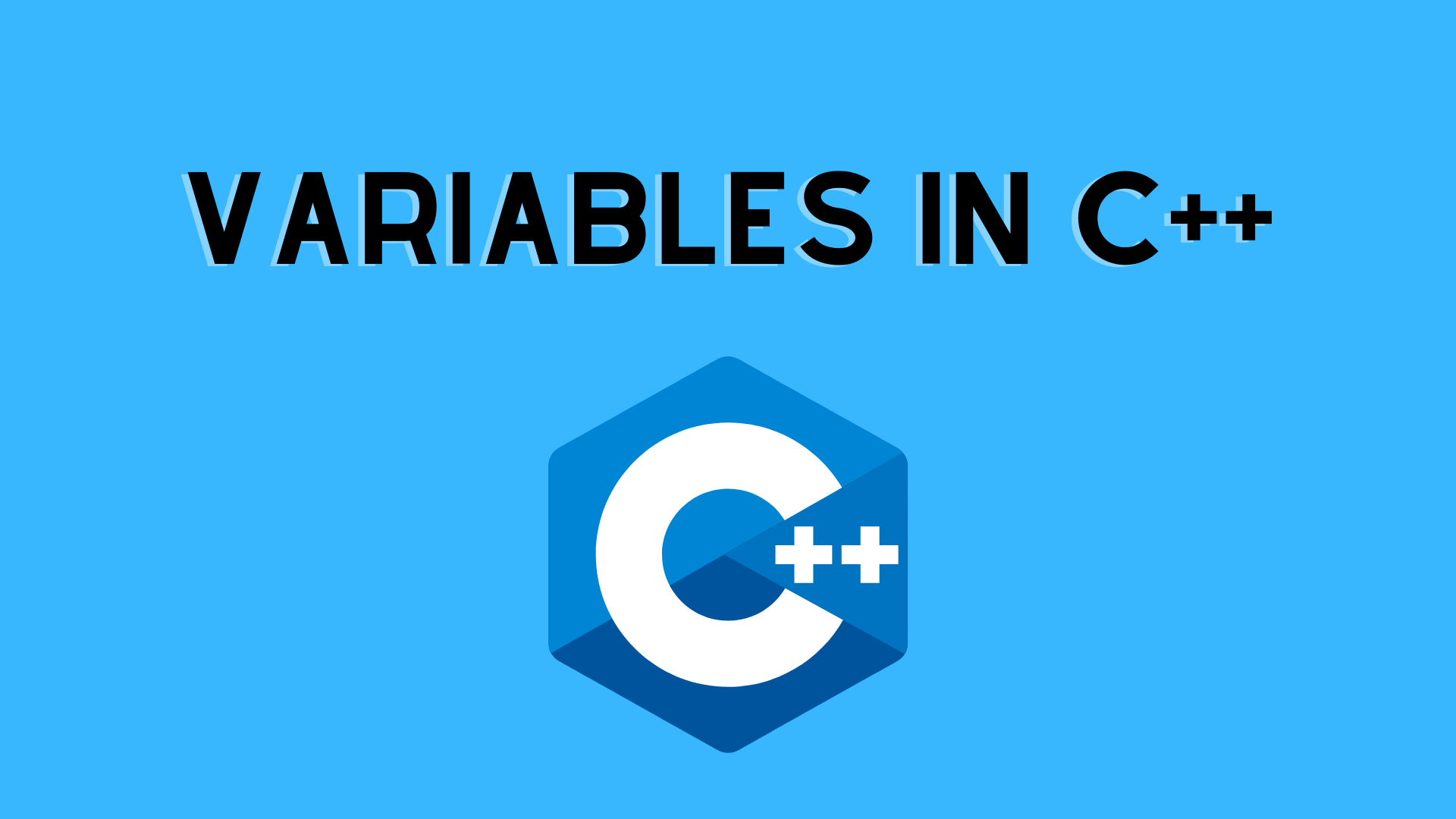## Variables in C++

In this tutorial, we will about variables in C++/ C  with an example.

variable is a type of named storage that our programs can access. The specific type of each variable in C++ determines the size and layout of the memory of the variable, the range of values that can be stored in that memory, and the set of operations that can be applied.

• Variables are data storage containers.
• The term “variable” refers to the name of a memory location.
• Each variable should have a unique name to indicate the storage area (identifier).
• A variable’s value can be updated while the programme is running.
• A variable is nothing more than a name for a memory place; anything you do with it affects that memory address.
• All variables in C++ must be declared before they may be used.

Example:

```int n = 5;
float = 5.99;
char = 'D';
boolean = true;
String = "Hello"; ```

Letters, numerals and the underscore character can all be used in a variable’s name. Either a letter or an underscore must be used as the first character. Because C++ is case-sensitive, upper and lowercase letters are separate.

There are three types of variables in C++:

### Variable Declaration in C++ : Example

A variable declaration assures the compiler that there is only one variable of the provided type and name, allowing the compiler to continue compiling without knowing all of the variable’s details. A variable declaration only has meaning when the program is being compiled; the compiler requires actual variable definition when the program is being linked.

When using multiple files, a variable declaration is handy because you can specify your variable in one of the files that will be available when the program is linked. You can declare a variable anywhere by using the extern keyword. Though a variable can be declared numerous times in a C++ program, it can only be defined once in a file, a function, or a block of code.

Example:

Consider the following example, in which a variable is declared at the top but not defined until the main function is called.

```#include <iostream>
using namespace std;

// Variable declaration:
extern int a, b;
extern int c;
extern float f;

int main () {
// Variable definition:
int a, b;
int c;
float fa;

// actual initialization
a = 10;
b = 20;
c = a + b;

cout << c << endl ;

f = 60.0/4.0;
cout << fa << endl ;

return 0;
}```

When the preceding code is compiled and run, the following result is obtained:

30
23.3333

variables in c++ variables in c++ variables in c++ variables in c++ variables in c++ variables in c++ variables in c++ variables in c++ variables in c++ variables in c++ variables in c++ variables in c++variables in c++variables in c++ variables in c++ variables in c++ variables in c++

## Lvalues and Rvalues

There are two kinds of expressions in C++:

• The term “lvalue” refers to expressions that refer to a memory location. An lvalue can be found on either the left or right side of an assignment.
• The term “rvalue” refers to a data value that is stored in memory at a specific address. An rvalue is an expression that can’t have a value given to it, hence it can only occur on the right side of an assignment, not the left.

Variables are lvalues, therefore they can appear on the assignment’s left side. Numeric literals are rvalues, which means they can’t be assigned and can’t display on the left. The following is an example of a valid statement:

int g = 20;

However, the following statement is not valid and will result in a compile-time error:

10 = 20;

You may like:

Features of C++ | C++ Tutorial

C++ Compiler